Previous column Next column

 The Theory of Classification Part 2: The Scratch-Built Typechecker Anthony J.H. Simons, Department of Computer Science, University of Sheffield, UKCOLUMN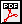PDF Version

1 INTRODUCTION

This is the second article in a regular series on object-oriented type theory, aimed specifically at non-theoreticians. Eventually, we aim to explain the behaviour of languages such as Smalltalk, C++, Eiffel and Java in a consistent framework, modelling features such as classes, inheritance, polymorphism, message passing, method combination and templates or generic parameters. Along the way, we shall look at some important theoretical approaches, such as subtyping, F-bounds, matching and the object calculus. Our goal is to find a mathematical model that can describe the features of these languages; and a proof technique that will let us reason about the model. This will be the "Theory of Classification" of the series title.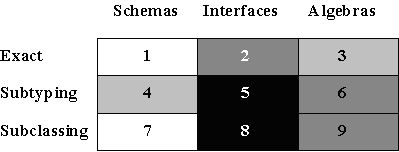Figure 1: Dimensions of Type Checking

The first article  introduced the notion of type, ranging from the programmer's concrete perspective to the mathematician's abstract perspective, pointing out the benefits of abstraction and precision. From these, let us choose three levels of type-checking to consider: representation-checking (bit-schemas), interface-checking (signatures) and behaviour-checking (algebras). Component compatibility was judged according to whether exact type correspondence, simple subtyping or the more ambitious subclassing was expected. Combining these two dimensions, there are up to nine combinations to consider, as illustrated in figure 1. However, we shall be mostly interested in the darker shaded areas. In this second article, we shall build a typechecker that can determine the exact syntactic type (box 2, in figure 1) of expressions involving objects encoded as simple records.

2 THE UNIVERSE OF VALUES AND TYPES

Rather like scratch-building a model sailing ship out of matchsticks, all mathematical model-building approaches start from first principles. To help get off the ground, most make some basic assumptions about the universe of values. Primitive sets, such as Boolean, Natural and Integer are assumed to exist (although we could go back further and construct them from first principles, in the same way as we did the Ordinal type ; this is quite a fascinating exercise in the *-calculus ). All other kinds of concept have to be defined using rules to say how the concept is formed, and how it is used. We shall assume that there are: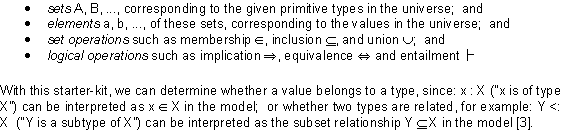3 RULES FOR PAIRS

An immediately useful construction which we do not yet have is the notion of a pair of values,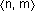, possibly taken from different types N and M. The type of pairs is known as a product type, or Cartesian product, since there are N x M possible parings of elements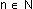, and. Formally, we require a rule to introduce the syntax for a product type. This is called a type introduction rule. In its simplest form (ignoring the notion of context, which is roughly the same idea as variable scope), the rule for forming a product is: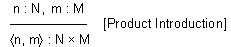This rule is expressed in the usual style of natural deduction, with the premises above the line and the conclusions below. In longhand, it says "if n is of type N and m is of type M, then we may conclude that a pairhas the product type N x M". The rule introduces the syntax for writing pair-values and pair-types, but also defines the relationship between the values and the types (the order of the values n and m determines the structure of the type N x M).

For pair constructions to be useful, we need to know how to access the elements of a pair, and determine their types. We define the first and second projections of a pair, usually written in the style: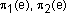applied to some pair-value e. The projections are defined formally in an elimination rule, so called because it deconstructs the pair to access its elements: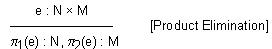"If e is a pair of the product type N x M, then the first projection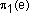has the type N, and the second projection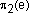has the type M." Note that, in this rule, e is presented as a single expression-variable, but we know it stands for a pair from its type N x M. In both rules, the horizontal line has the force of an implication, which we could also write using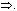4 RULES FOR FUNCTIONS

Consider an infinite set of pairs: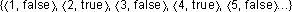. This set is an enumeration of a relationship between Natural numbers and Boolean values - it is in fact one possible representation of the function even(). Since functions have this clear, natural interpretation in our model, we are justified in introducing a special syntax for them: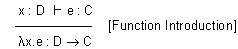"If variable x has the type D and, as a consequence, expression e has the type C, we may conclude that a function of x with body e has the function type D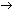C." This rule introduces the-syntax for functions and the arrow-syntax for function types. If you happen to be a hellenophobic1 engineer, simply consider that:x.e * f(x)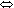e. The type signature for a function is always written as an arrow type DC, with the function's domain (input set) D on the left and the codomain (output set) C on the right. The premise of this rule relates the required types of x : D, e : C using entailment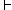, since the type of the body expression e : C is not independent, but "follows from" the type of the variable x : D. This is because the body expression will contain occurrences of x, the variable. Consider that a function may return its argument - in that case, the result type is the argument type; there is clearly a dependency.

The function elimination rule explains the type of an expression involving a function application. In so doing, it also defines the parenthesis syntax for function application: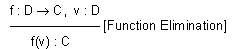"If f is a function from DC, and v is a value of type D, then the result of the function application f(v) is of type C". This rule also expresses the notion of type-sound function application: it allows f to be applied only to values of the domain type D (technically, the rule allows you to deduce that the result of function application is well-typed in this circumstance, but is otherwise undefined).

Do we need rules for multi-argument functions? Not really, because we already have the separate product rules. The domain D in the function rules could correspond, if we so wished, to a type that was a product: DN x M. In this case, the argument value would in fact be a pair v : N x M. We assume that any single type variable in one rule can be matched against a constructed type in another rule, if we so desire.

5 RULES FOR RECORDS

Most model encodings for objects [4, 5, 6] treat them as some kind of record with a number of labelled fields, each storing a differently-typed value. So far, we do not have a construction for records in our model. However, consider that a record is rather like a finite set of pairs, relating labels to values: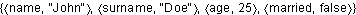. Since a record has this clear, natural interpretation in the model, we are justified in introducing a special syntax. If A is the primitive type of labels: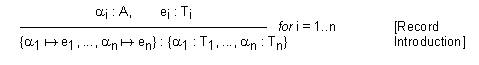"If there are n distinct labels, and n values ei of different corresponding types Ti then a record, constructed by pairing the ith label with the ith value, has a record type, which is constructed by pairing the ith label with the corresponding ith type". This rule uses the index i to link corresponding labels, values and types. In the conclusion, the set-braces are used for records and record types deliberately, since these are sets of pairs. The label-to-value pairings are notated as mappings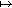ei, for visual clarity and convenience.

The corresponding record elimination rule introduces the dot or record selection operator, defining how to deconstruct a record to select a field and then determine its type: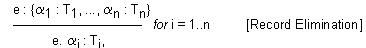"If e has the type of a record, with n labels, mapping to types Ti, then the result of selecting the ith field e.has the ith type Ti."

6 APPLYING THE RULES

We have a set of rules for constructing pairs, functions and records. With this, we can model simple objects as records. Ignoring the issue of encapsulation for the moment, a simple Cartesian point object may be modelled as a record whose field labels map to simple values (attributes) and to functions (methods). We require a function for constructing points:

make-point : Integer x IntegerPoint

This is a type declaration, stating that make-point is a function that accepts a pair of Integers and returns a Point type (which is so far undefined). The full definition of make-point: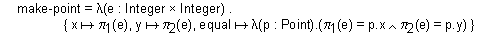names the argument expression e supplied upon creation and returns a record having the fields x, y and equal. The x and y fields map to simple values, projections of e; the equal field maps to a function, representing a method. Note that make-point is built up in stages according to the type rules. The product introduction rule can construct a pair type: Integer x Integer from primitive Integers. The function type of equal: PointBoolean can be inferred from the type Point supplied as the argument, and the type of the body expression, using the function introduction rule: the body is a logical "and"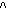expression, a primitive Boolean operation provided with the starter-kit. The record type Point is the type of the value returned by make-point. Using the record introduction rule, we determine that this is equivalent to a record type: { x : Integer, y : Integer, equal : PointBoolean }, by examining the individual types of the label-value pairs supplied as its fields. Finally, even make-point is properly constructed using the function introduction rule, with Integer x Integer as the domain type and Point as the codomain type.

The rules permit us to deduce that objects can be constructed using make-point, and also that they are well-typed. Let us now construct some points and expressions involving points, to see if these are well-typed. The let ... in syntax is a way of introducing a scope for the values p1 and p2, in which the following expressions are evaluated:

 letp1 = make-point(3, 5) in p1.x;

Is p1.x meaningful, and does it have a type? The record elimination rule says this is so, provided p1 is an instance of a suitable record type. Working backwards, p1 must be a record with at least the type: {... x : X ... } for some type X. Working forwards, p1 was constructed using make-point, so we know it has the Point type, which, when expanded, is equivalent to the record type: { x : Integer, y : Integer, equal : Point * Boolean }, which also has a field x : Integer. Matching up the two, we can deduce that p1.x : Integer.

 let p1 = make-point(3, 5), p2 = make-point(3, 7) inp1.equal(p2);

Is p1.equal(p2) meaningful, and does it have a type? Again, by working backwards through the record elimination rule, we infer that p1 must have at least the type {... equal : Y ...} for some type Y. Working forwards, we see that p1 has a field equal : PointBoolean, so by matching up these, we know YPointBoolean. So, the result of selecting p1.equal is a function expecting another Point. Let us refer to this function as f. In the rest of the expression, f is applied to p2, but is this type correct? Working forwards, p2 was defined using make-point, so has the type Point. Working backwards through the function elimination rule, the function application f(p2) is only type-sound if f has the type PointZ, for some type Z. From above, we know that f : PointBoolean, so by matching ZBoolean, we confirm that the expression is well-typed and also can infer the expression's result type: p1.equal(p2) : Boolean.

7 CONCLUSION

We constructed a mathematical model for simple objects from first principles, in order to show how it is possible to motivate the existence of something as relatively sophisticated as an object with a (constant) state and methods, using only the most primitive elements of set theory and Boolean logic as a starting point. The type rules presented were of two kinds: introduction rules describe how more complex constructions, such as functions and records, are formed and under what conditions they are well-typed; elimination rules describe how these constructions may be decomposed into their simpler elements, and what types these parts have. Both kinds of rule were used in a typechecker, which was able to determine the syntactic correctness of method invocations. The formal style of reasoning, chaining both forwards and backwards through the ruleset, was illustrated. The simple model still has a number of drawbacks: there is no updating or encapsulation of state; there are problems looming to do with recursive definitions; and we ignored the context (scope) in which the definitions take effect. In the next article, we shall examine some different object encodings that address some of these issues.

Footnote
1Hellenophobe: a hater of Greek symbols

REFERENCES

 A J H Simons, Perspectives on type compatibility, Journal of Object Technology 1(1), May, 2002.

 A J H Simons, Appendix 1 : *-Calculus, in: A Language with Class: the Theory of Classification Exemplified in an Object-Oriented Language, PhD Thesis, University of Sheffield, 1995, 220-238. See http://www.dcs.shef.ac.uk/~ajhs/classify/.

 L Cardelli and P Wegner, On understanding types, data abstraction and polymorphism, ACM Computing Surveys, 17(4), 1985, 471-521.

 J C Reynolds, User defined types and procedural data structures as complementary approaches to data abstraction, in: Programming Methodology: a Collection of Articles by IFIP WG2.3, ed. D Gries, 1975, 309-317; reprinted from New Advances in Algorithmic Languages, ed. S A Schuman, INRIA, 1975, 157-168.

 W Cook, Object-oriented programming versus abstract data types, in: Foundations of Object-Oriented Languages, LNCS 489, eds. J de Bakker et al., Springer Verlag, 1991, 151-178.

 M Abadi and L Cardelli. A Theory of Objects. Monographs in Computer Science, Springer-Verlag, 1996.Anthony Simons is a Senior Lecturer and Director of Teaching in the Department of Computer Science, University of Sheffield, where he leads object-oriented research in verification and testing, type theory and language design, development methods and precise notations. He can be reached at a.simons@dcs.shef.ac.uk